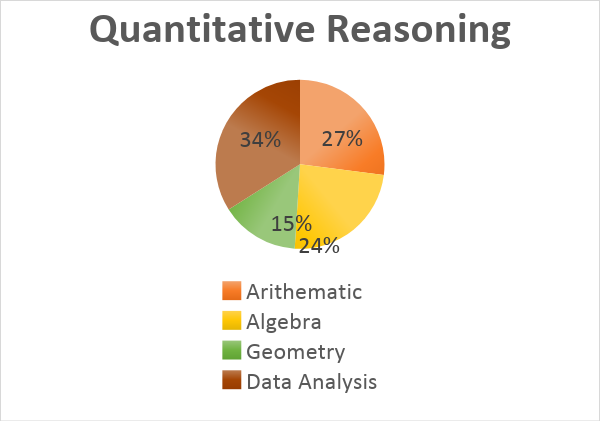# Breakdown of GRE Quantitative Concepts

GRE exempts you from the dreaded higher-level math like calculus and trigonometry. Didn’t that take the heat off already? The quantitative section, however, tests you on four areas of math. It’s a push for your basic mathematical skills as the test gauges your ability to interpret and analyze quantitative information. Overall, you have two twenty-question-sections that come with a time frame of 35 minutes. On a lighter note, 40 questions in 70 minutes! Here is a breakdown of four sections covered by quantitative reasoning-The question type, for the most, is MCQ’s but look out for numeric entry problems where you do not have the leverage of plugging answer choices to simplify expressions. Data analysis questions being the heart of the quantitative reasoning requires you to be good at statistical measurements. Quantitative comparisons can be solved in well under 60 seconds. Banking time while solving comparison questions allow you to spend more time on tricky data interpretation questions, such as tables and charts.

A couple of reminders-

1. Variables in comparison problem can be a negative integer, a positive integer, a fraction or a zero– don’t ever make assumptions!
2. Unlike geometric figures like triangles, circles, and quadrilaterals, graphical data or statistics are drawn to scale and can be estimated visually.

A detailed split up of each section is as follows-

Arithmetic

Algebra

Geometry

Data analysis

Prime Numbers & Factorization

Sequences

Number Lines

Exponents & roots

Percentage & Ratios

Direct & Inverse Proportionality

Absolute Value & Modulus

Fractions, Decimals & Digits

Number sequences

Functions

Equalities & inequalities

Graphs &Intercepts

Slopes

Parallel & Perpendicular lines

Congruent & similar figures

Area, perimeter, volume

3D geometry

Coordinate Geometry

Probabilities

Frequency distributions

Line graphs

Bar graphs

Histograms

Circle graphs

Box plots

Scatter plots

Counting Methods

The GRE allows the use of a calculator in the math section, and it comes handy when you are unsure about your mental computation. A quick guideline for calculator use is as follows-

1. Check the order of operations of the on-screen display. It might vary from your basic calculator.
2. Use transfer-display button to transfer the result to the answer box in the numeric entry problem.
3. If your answer choices are a fraction, avoid the calculation as the calculator displays decimals in place of a fraction.

Most problems can be answered more quickly by estimating rather than by using the calculator. So, use it when you really need it! Consider making the quantities look similar by manipulating either of the two to save both time and effort. Also, word problems can be your score booster if and only if you stop and ask yourself- Did I use all the necessary information and adhere to the condition of the problem?